# Recall: Rotation matrices

Let’s quickly recall rotation matrices used to rotate a point in Euclidean space by an angle θ about the x-, y- or z-axis. We deduce the rotation matrix for the x-axis visually. First, consider this coordinate system with a point P living on the yz-plane as depicted below.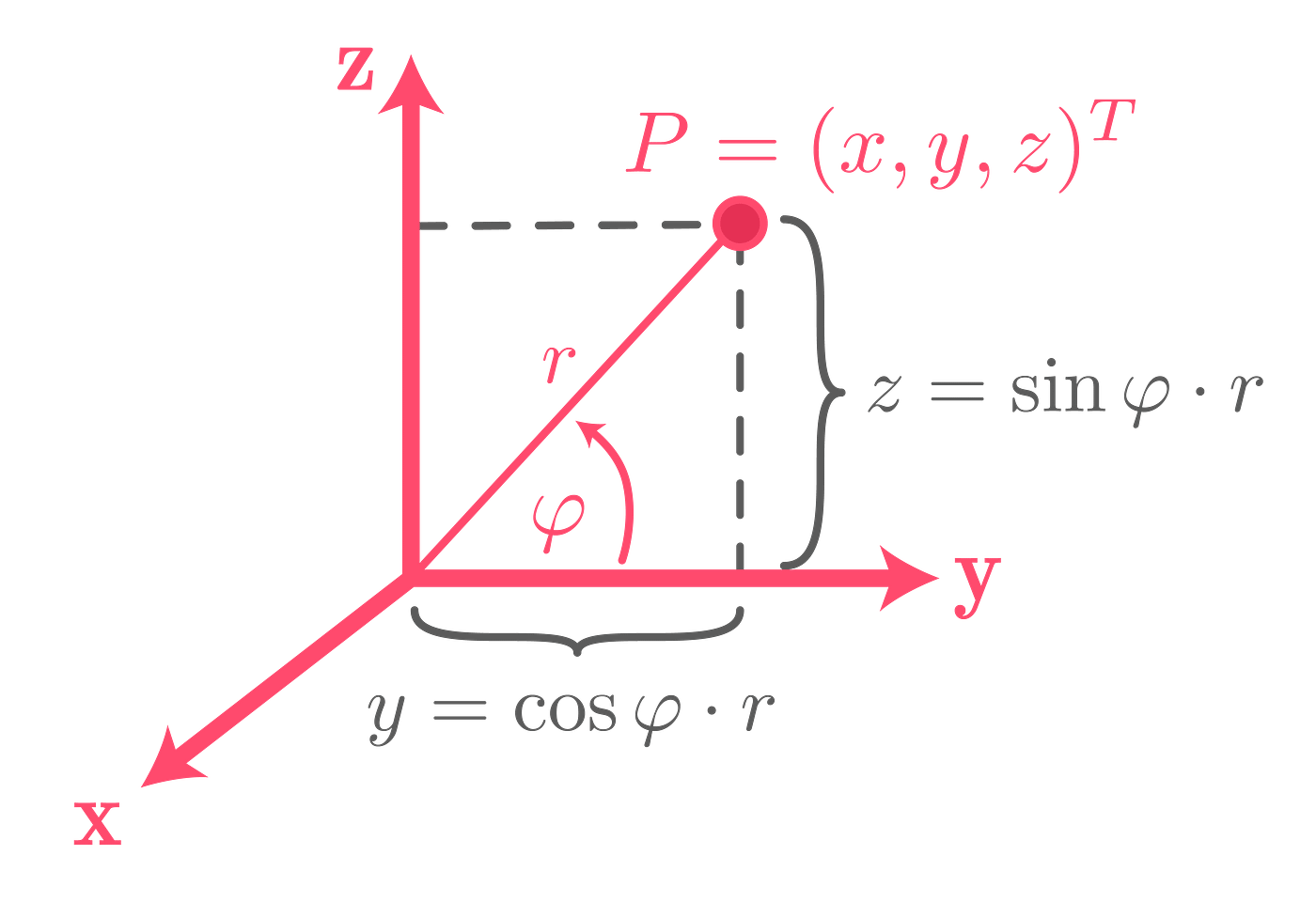Point P in a coordinate system

We can express the coordinates of this point in terms of an angle φ and a length r from the origin. Now, take a look at the coordinate system after having applied a positive rotation by angle θ about the x-axis.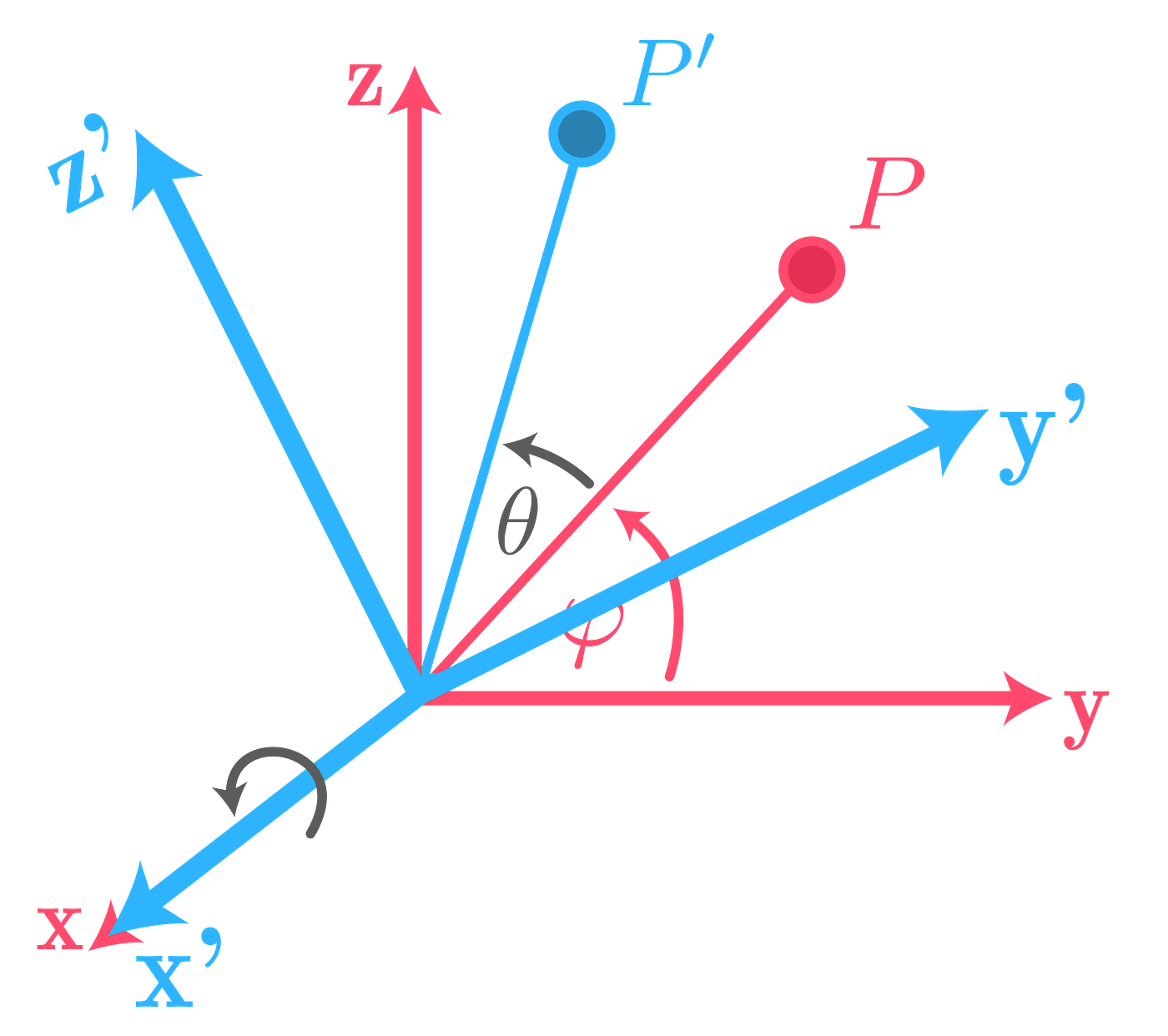Point P’ in the rotated coordinate system

We can express the new point in terms of an angle φ + θ and r, use angle sum identity for sine and cosine and finally get: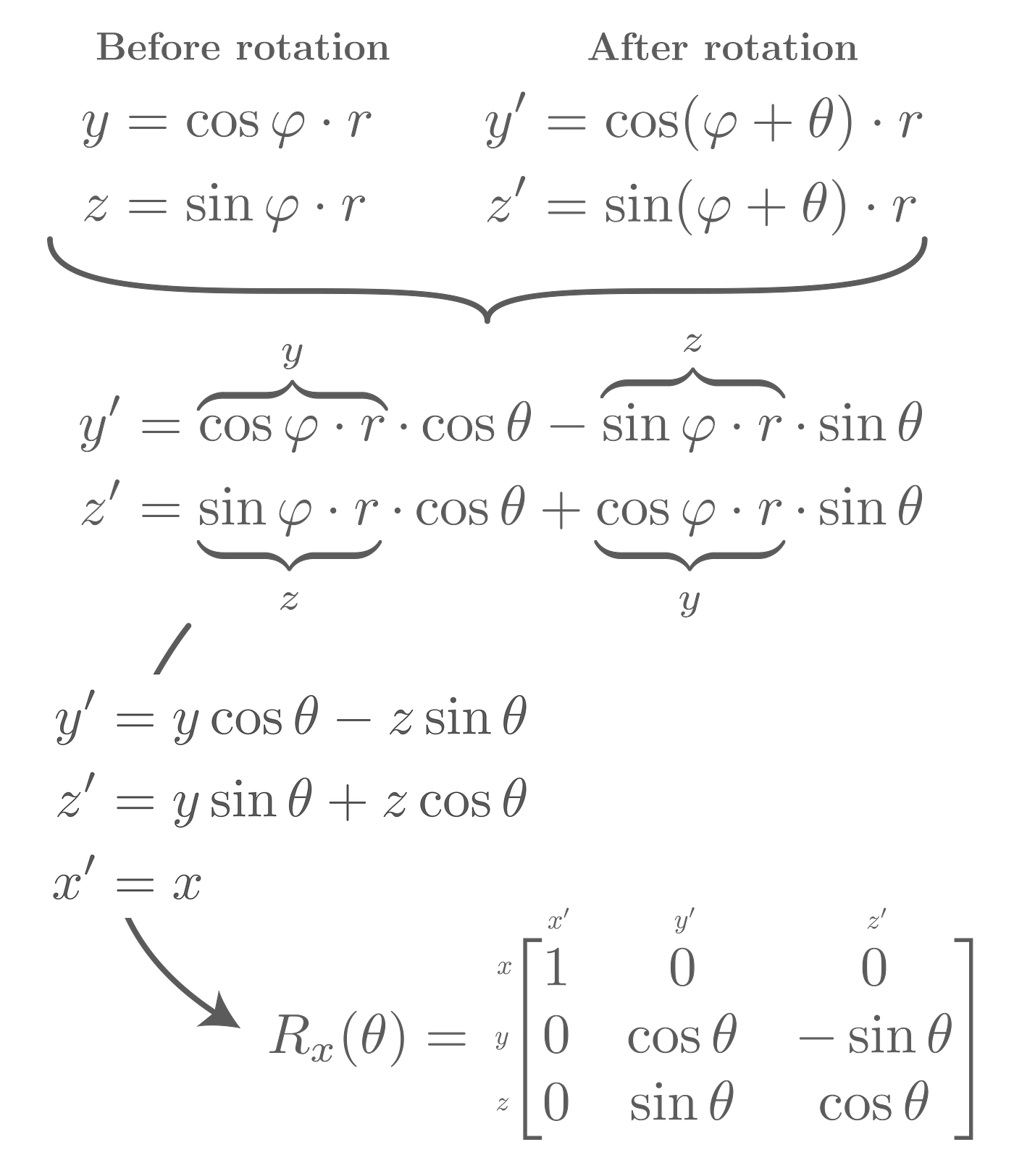Derivation of the rotation matrix for rotation by θ about the x-axis

Tada, we’ve derived the rotation matrix for a rotation about the x-axis. You can deduce the other rotation matrices (for the y- and z-axis) similarly.

# Extrinsic & intrinsic rotations

Multiplying two of our rotation matrices together yields another matrix that combines those two rotations into a single one. The question that arises is whether the second, third, … rotations all refer to our global coordinate system (extrinsic) or to the last rotated coordinate system. To state it clearly:

• extrinsic: rotations all refer to a fixed/global coordinate system xyz
• intrinsic: a rotation refers to the last rotated coordinate system (starting with the first rotation that refers to the original/global coordinate system)

Take the example of an airplane. We can rotate about one of its three axes. Instead of using greek letters for the angles, a common convention (air norm DIN 9300) is to call them yaw, pitch and roll angle.

Let’s chain these elemental rotations together. First, yaw the airplane in the positive direction (to the right) by 90°, then pitch it in the positive direction (upwards) by 45° and roll by 180° in the positive direction (to the right).

The rotated frame is marked in blue. Initially, it is aligned with our global coordinate system marked in red. After the 90° yaw, the 45° pitch is applied to the rotated blue frame and not to the red one. Hence, this sequence of rotations is intrinsic. It is often denoted by z-y’-x’’ as we are rotating about the original z-axis, a new y-axis and finally a new x-axis. We can easily derive the resulting rotation matrix by looking at where our unit vectors, spanning the base of the global coordinate system, land after having applied all three rotations.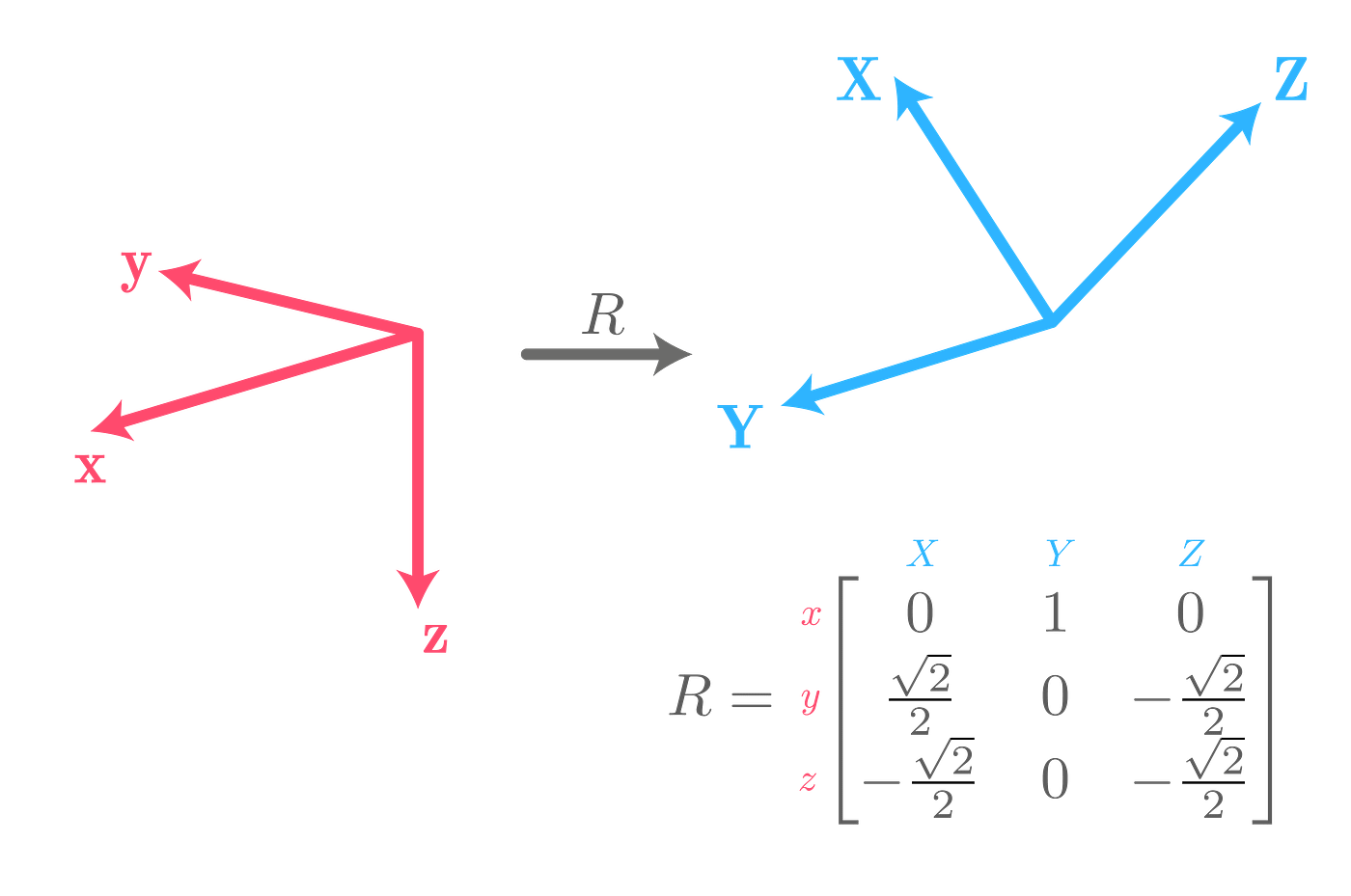Yaw-Pitch’-Roll’ rotation matrix (consistent with the airplane animation above)

We’ve transformed our global/world coordinate system xyz into the XYZ coordinate system, also called body frame since it is attached to our body (our airplane). The new Y unit vector has landed on the position of the old x unit vector, therefore the column in the rotation matrix reads as (1, 0, 0). X is composed of a multiple of the old y- and the old z vector. As X is pointing upwards we have to walk along the red z-axis in the negative direction and the same amount in the positive y direction to arrive at the position of the new, blue X vector. Note that sine and cosine of 45° is √2/2, so this coefficient stems from our 45° rotation for the pitch. You can deduce the last column for Z likewise (I leave that for you).

How do we calculate R using our rotation matrices instead of looking at the final position of the unit vectors? We chain linear transformations (represented by matrices) through multiplication. Matrix multiplication is not commutative, so the question is in which order we multiply the elemental rotations. You know the rotation matrices, so you can try this out on your own. Only the following order of operands will yield the correct rotation matrix you’ve seen above.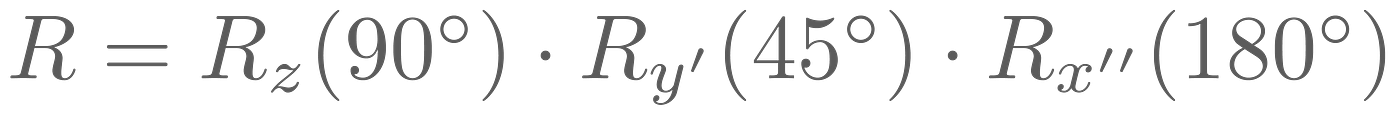Multiplication of elementary rotations. Prime symbols are just used to clarify that we rotate about new axes. In fact, R_y’ is the exact same rotation matrix as R_y.

We will answer the Why is this the case? in the end. For the moment, you can at least confirm that this calculation is correct by calculating the multiplication by hand.

Now, let’s take a look at the corresponding extrinsic sequence of rotations that places the airplane in the exact same position as beforehand. However, instead of rotating about the body frame (blue) that changes its orientation after each elemental rotation, we now always rotate with respect to a fixed/global/world coordinate system (red).

The end position of our airplane is exactly the same as it was previously! From this we can infer that the rotation matrix R must also have remained the same. However, to get there, the order of our steps has changed: instead of a Yaw-Pitch’-Roll’’ (z-y’-x’’) sequence of rotations, we’ve used Roll-Pitch-Yaw (x-y-z), so we first applied roll (about the global x-axis), then pitch (about the global y-axis) and finally yaw (about the global z-axis). Notice the lack of the prime symbol indicating that we always refer to our global coordinate system xyz. Thinking in terms of this fixed frame, what is the order of arguments for the multiplication now? Well… it is once again:Multiplication of elementary rotations (once more)

There is only this order of matrices yielding the desired rotation matrix that combines all three rotations into one. What’s the big deal then? Confusion might arise when we summarize the differences between the two calculations:

• Our intrinsic example: Yaw-Pitch’-Roll’’ (z-y’-x’’), that is,
1) rotation about the global z-axis
2) rotation about the new y’-axis
3) rotation about the new x’’-axis
Matrix multiplication: R = Rotation1 ⋅ Rotation2 ⋅ Rotation3
• Our extrinsic example: Roll-Pitch-Yaw (x-y-z), that is,
1) rotation about the global x-axis
2) rotation about the global y-axis
3) rotation about the global z-axis
Matrix multiplication: R=Rotation3 ⋅ Rotation2 ⋅ Rotation1

We’ve swapped both the order of steps to transfer our original coordinate system xyz into a new, rotated system XYZ as well as the order of arguments for the matrix multiplication. This is the reason why we end up with the same rotation matrix R. We either talk about rotating several times about a fixed reference frame, or one that changes with each rotation. Personally, I am skeptical about the expression “multiply from the right or from the left”, but if you insist, you could conclude that for the intrinsic sequence of rotations, we multiply from left to right, while we multiply from right to left for the extrinsic case.

# Bonus: Why is the order of arguments like this?

It’s pretty astonishing that we are able to arrive at the same goal making use of this duality. So far, we’ve motivated the order of matrices for the multiplication by calculation and since “it just doesn’t work the other way around”. Let’s take another, closer look here.

The extrinsic case should seem like the “normal” case in terms of matrix multiplication where you read from right to left. This becomes clearer when we try to transform a point with this rotation matrix: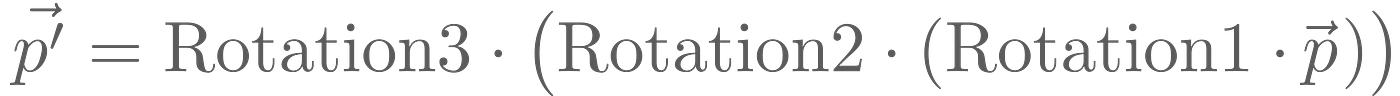Rotate point P (vector p) to Point P’ (vector p’)

We rotate our original global coordinate system, using the Rotation1 matrix. Then, rotate the resulting system using the Rotation2 matrix and finally using our third rotation matrix. Of course, matrix multiplication is associative, so the parentheses are just there to make the statement clear: when we want to transform a point, we read from right to left and thus first apply Rotation1, then Rotation2 and finally Rotation3. If you feel uncertain about this, definitely check out this video by 3Blue1Brown.

So, the extrinsic case (R=Rotation3⋅ Rotation2⋅ Rotation1) should make sense to you. But what about the intrinsic sequence of rotations, where everything is happening in reverse: R = Rotation1⋅ Rotation2 ⋅ Rotation3. If we were to transform a point, we would first apply Rotation3, then Rotation2, then Rotation1 and end up with our transformed point P’. This seems to contradict with the order of steps defined beforehand for the intrinsic sequence of rotations. To clear up this confusion, we have to clearly indicate in relation to which coordinate system we are specifying the coordinates of a point P. We introduce the following new syntax.

By this, we express the coordinates of point P with respect to our original coordinate system. The following expression

describes the orientation of coordinate system A with respect to the original coordinate system. In other words, matrix R times a unit vector of Original gives the position of the respective unit vector of A with respect to the original coordinate system. Let’s see this in action and rotate the unit vector
P=(1, 0, 0) by +45° about the z-axis.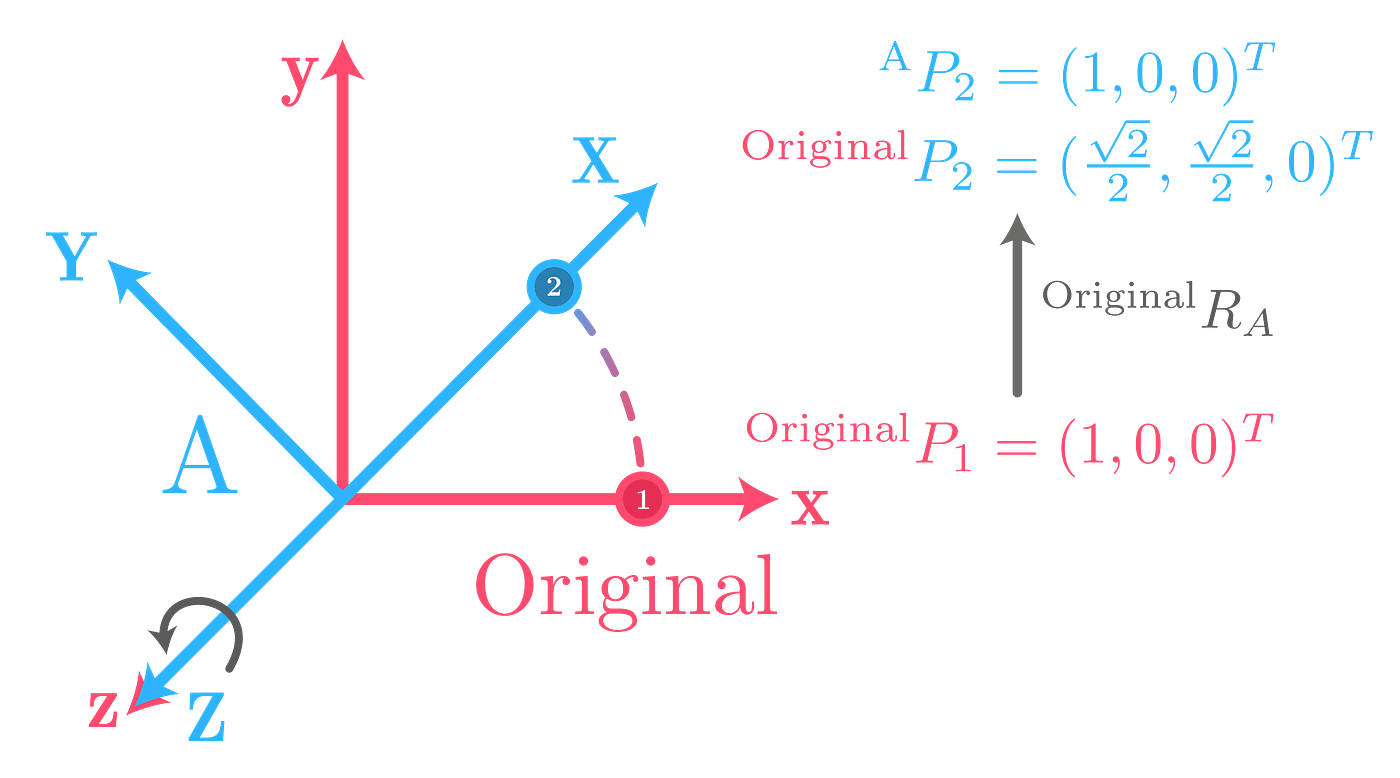Rotating by 45° about the z-axis. Points 1 and 2 in different reference systems.

Point P₂ is expressed in two variants: with regards to the original coordinate system and in relation to the newly rotated, blue coordinate system A. The key is that P₂ in terms of A has the same coordinates as P₁ in terms of the original coordinate system. Thus, when we apply the rotation matrix R to the point P₂ expressed in terms of A — which is (1,0,0) — we retrieve the point (√2/2, √2/2, 0) — which is the same point in terms of the original coordinate system. Thus, we “converted” the coordinates of point P₂ from frame A to the original frame. This idea is crucial, so let’s illustrate it.

This emphasizes that we have to separate the construction of our matrix R from its actual application when transforming a point:

• Left path — Construction of matrix R: Rotate the original coordinate system to coordinate system A. This way, point P₁ is rotated to P₂, both with respect to original.
• Right path — Application of matrix R: Given a point in terms of coordinate system A, we can “convert” its coordinates to the original coordinate system and we do this by multiplying with the same matrix that we constructed by rotating original into A. This is the crux where all the “inverted-reverse-headspinning-multiplications” come from for the intrinsic sequence of rotations. Notice, that the right path yields the following formula. You can see that the A as subscript is “cancelling out”.

Finally, we bring everything together. Let’s see the chaining of rotations in action with our new syntax. We rotate by 45° about the z-axis, and then again by 45° about the z’-axis (which happens to coincide with the z-axis).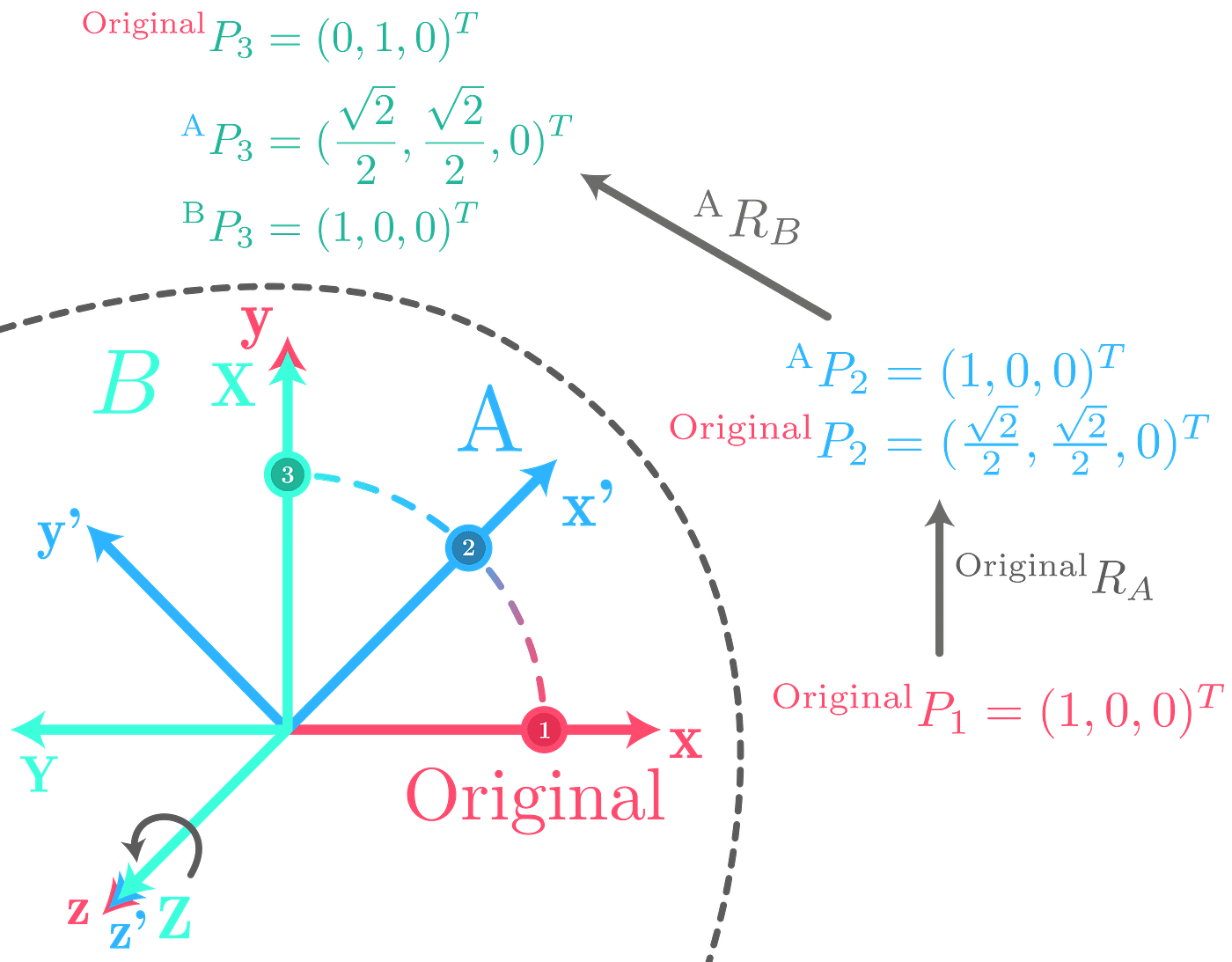Two chainedrotations: 1) 45° about the z-axis 2) 45° about the z’ axis

Again, illustrate the role of the different rotation matrices.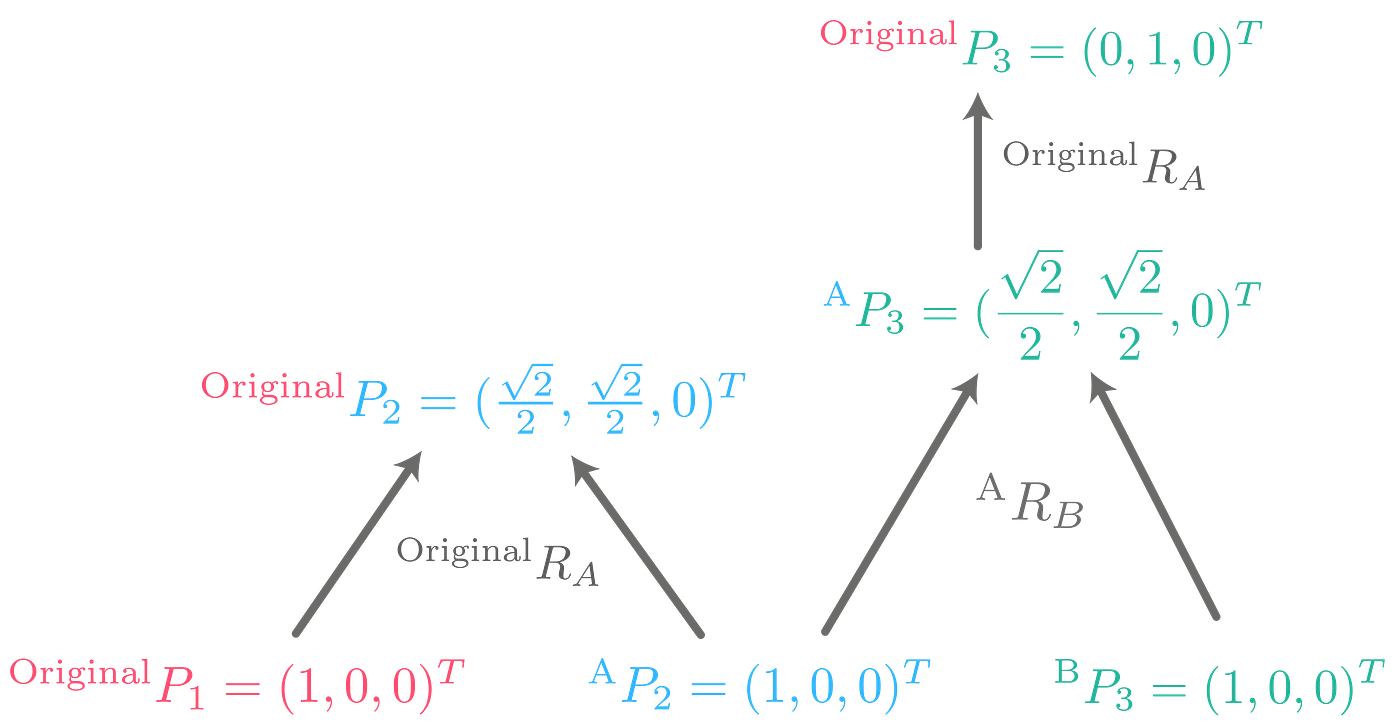“Conversion” between different reference systems with two rotations (paths to the right)

So, what is this telling us? Think of the following exercise: You have an original coordinate system xyz and rotate it by 45° about the z-axis to get coordinate system A. Then, with reference to A (this means: intrinsic), rotate about its z-axis again by 45° to get coordinate system B. Your task: given a point with respect to B, what are its coordinates with respect to the original coordinate system?

How do we solve this problem? Well, let’s look at our graph. In the bottom-right corner, we see a point with respect to B. How do we “convert” to its original coordinates? Simply walk along the edges. First, multiply with the rotation matrix that defines where B is in terms of A. By doing so, we know where P₃ is in terms of A. Then multiply with the rotation matrix that defines where A is with respect to the original coordinate system. By doing so, we know where P₃ is with respect to the original coordinate system. That’s it.

Now, compare this to what we’ve done in the first half. How do we construct the rotation matrix that combines these two rotations (intrinsic)? Just trace where the coordinate systems go, starting with the original coordinate system xyz and ending with the rotated system XYZ. Along the way, chain the matrices from left to right as you can see in the above formula. By doing so, when you apply this matrix, it’s as if you “unwinded” from B, then to A and then back to the original coordinate system.

And for the extrinsic case? The matrix multiplication stays the same; you read from right (first rotation) to left (last rotation). But in contrast to the intrinsic sequence of rotations, there is no need to “unwind” anything. The first step is therefore to go from the original coordinate system to A, then from A to B. In the matrix multiplication, the first step is far right, then comes the next step to the left. Therefore, here you chain matrices from right to left. That’s the difference.

Summary / Another way to tackle this: don’t remember whether you have to multiply going from right to left or from left to right. Instead, always think in terms of a point that you want to transform, that is, always chain matrices to the left of your point like you’re used to in linear algebra.
Then, the extrinsic case is easy: follow the coordinate systems as they rotate and apply the rotations (multiply by definition from right to left). Reference for each rotation is the global coordinate system.
The intrinsic case is also easy: for the extrinsic sequence of rotations, you’ve followed the coordinate systems from the first to the last rotation, here you do the opposite and “unwind” from the last coordinate system to the first one (multiply by definition from right to left).Reference for each rotation is the last rotated coordinate system.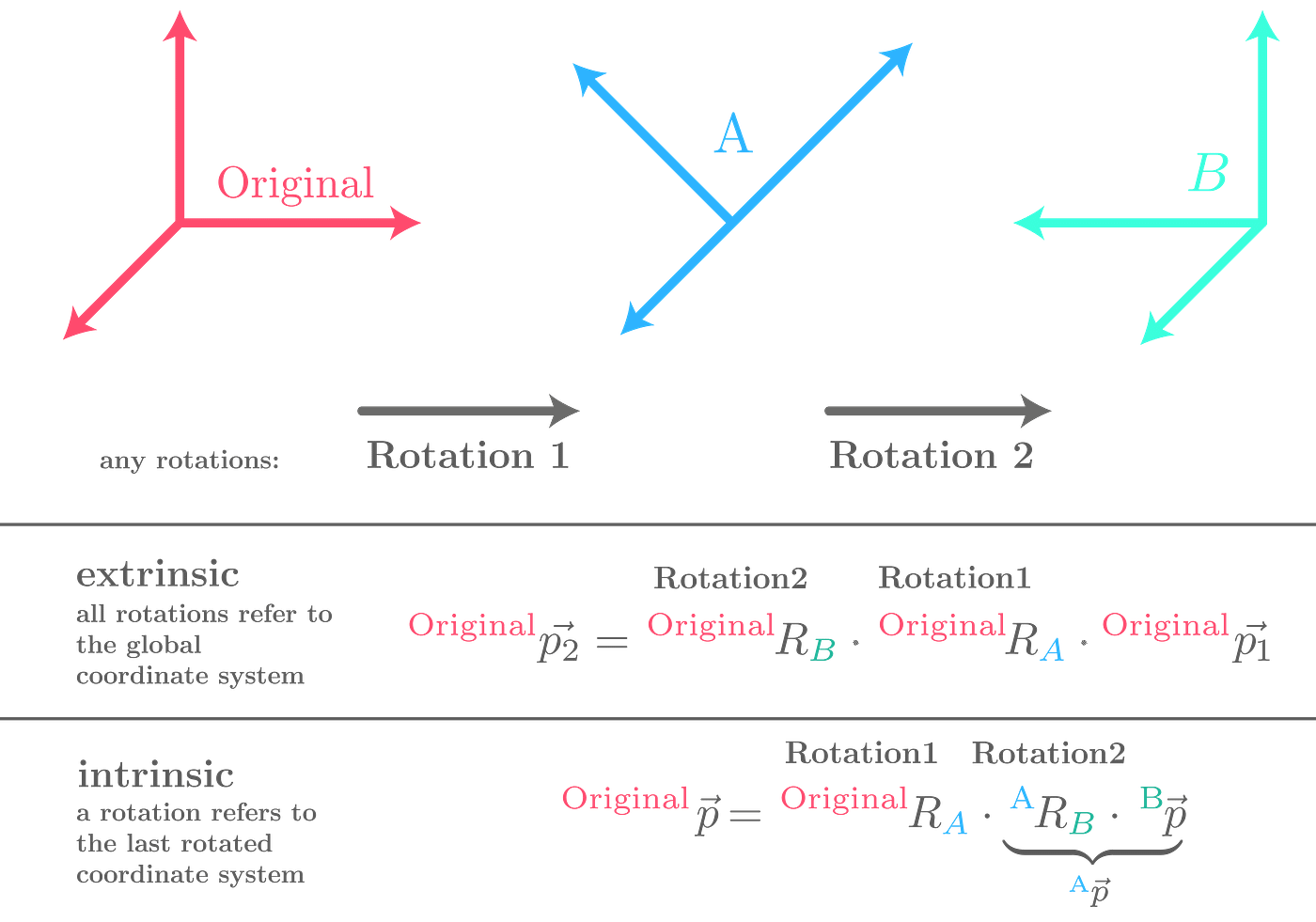Summary / Comparison of extrinsic and intrinsic sequence of rotations

# Wrapping up

In this post, we’ve first recalled rotation matrices including the derivation of the rotation matrix for rotation about the x-axis. We then compared extrinsic and intrinsic rotation by means of an airplane and figured out their duality. In the end, we made a deeper dive and answered the question, why the order of matrices when multiplied together is the way it is.

Although the math behind all of this is comparatively easy, grasping the concepts and thinking in terms of different reference systems is kind of hard. I struggled a lot and made a lot of mistakes with the sub- and superscripts upon designing the graphics. Hopefully, I ruled out all mistakes, but that’s probably not the case. So feel free to leave a comment if you spotted an error and I will gladly correct it. I hope that my post clarified some things for you and gave you a better intuition for this interesting application of linear algebra.

--

--## Dominic Plein

Fond of explaining things visually and getting creative through music (piano), math & computer science (currently a bachelor student).